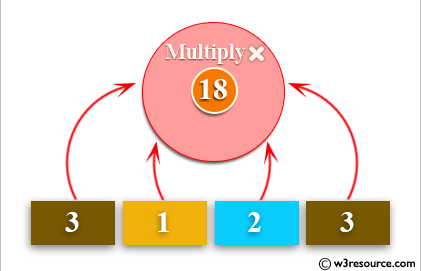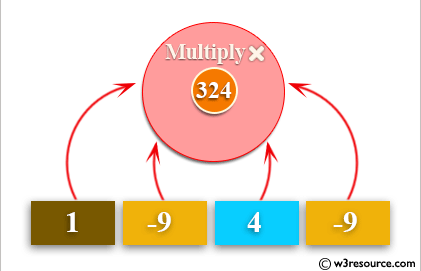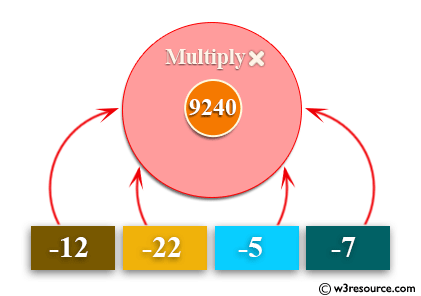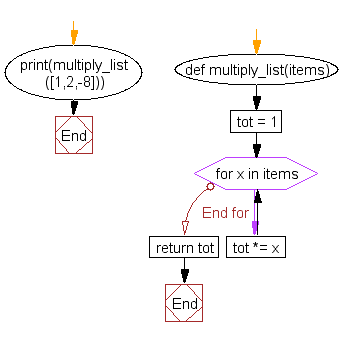﻿ Python: Multiplies all the items in a list - w3resource# Python: Multiplies all the items in a list

## Python List: Exercise-2 with Solution

Write a Python program to multiplies all the items in a list.

Example - 1 :Example - 2 :Example - 3 :Sample Solution:-

Python Code:

``````def multiply_list(items):
tot = 1
for x in items:
tot *= x
print(multiply_list([1,2,-8]))
```
```

Sample Output:

```-16
```

Flowchart:## Visualize Python code execution:

The following tool visualize what the computer is doing step-by-step as it executes the said program:

Python Code Editor:

Have another way to solve this solution? Contribute your code (and comments) through Disqus.

What is the difficulty level of this exercise?

Test your Python skills with w3resource's quiz

﻿

## Python: Tips of the Day

Checks if the given number falls within the given range.

Example:

```def tips_range(n, start, end = 0):
return start <= n <= end if end >= start else end <= n <= start
print(tips_range(2, 4, 6))
print(tips_range(4, 8))
print(tips_range(1, 3, 5))
print(tips_range(1, 3))
```

Output:

```False
True
False
True
```